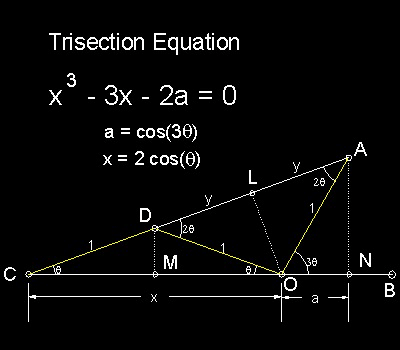History of the Problems
Go to   Fun_Math Content Table   Trisecting an Angle   Classical Solutions

### Classical Solutions

#### Trisection Equation

Archimedes' Solution is shown in the figure shown below.#### Derivation:

Algebraic Formulation:

Since three triangles CDM, COL and CAN are similar. CM/CD = CL/CO = CN/CA.

So x/2 = (1 + y)/x = (x + a)/(1 + 2y)

which gives ---> x2 = 2 + 2y and 1 + 2y = 2(x + a)/x

Eliminating y from these two equations, we obtain x 2 - 1 = 2(x + a)/x or

x 3 - 3x - 2a = 0

where x = 2cos(q) and a = cos(3q)

******** trisection_equation_desc.dwg *********

#### Trigonometric Formulation:

Using the trigonometric identities, cos(a+b) = cos(a)cos(b) - sin(a)sin(b) and sin(2a) = 2*sin(a)cos(a)

cos(3q) = 4cos3 (q) - 3 cos (q)

Using the relations (Refer to the figure); x = 2cos(q) and a = cos(3q)

This equation can now be written as

x 3 - 3x - 2a = 0

#### Note about derivation from Trigonometry

From the figure ; CB = CO + ON = CA * cos(q) = (CD + DA)cos(q)

Therefore a + x = (1 + 2y)*cos(q) , and y = DO *cos(2q)

From these relations, the same trisection equation can be derived.

#### References

1. Yates,Robert Carl : "The Trisection problem"

Go to   Fun_Math Content Table   Trisecting an Angle   Classical Solutions

All questions/suggestions should be sent to Takaya Iwamoto

Last Updated Nov 22, 2006